Next: 3.3.5 Effective Density of Up: 3.3 Band-Structure Previous: 3.3.3 Bandgap Narrowing

## 3.3.4 Effective Carrier Mass

In this work, parabolic conduction and valence bands are assumed, and the effects of band non-parabolicity are assumed to be negligible. Therefore, effective masses for each of the three conduction band minima (, X, and L) can be defined and used to calculate the density of states (DOS). The DOS in the valence band can be characterized by the effective masses of three sub-bands, all in the center of the Brillouin zone: two degenerate sub-bands of light and heavy holes, and the split-off sub-band. The contribution of the split-off band, which is depressed in energy and is much less populated, is neglected in this work.

The effective DOS masses scaled by the free electron mass give relative carrier masses. In materials with direct bandgap the value of the relative mass for electrons from thevalley is considered. In the case of materials with indirect bandgaps namely Si, Ge, AlAs, and GaP the transverse and the longitude electron masses (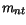and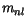) from the X valley (respectively L valley for Ge) are taken into account by (3.86). For all materials the hole masses are calculated by (3.87) accounting for the heavy and the light hole masses (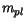and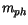) .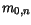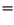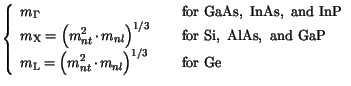(3.86)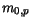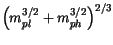(3.87)

The model parameters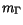,,,, andare summarized in Table 3.16. In addition, the calculated values for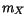andare included, after comparison to calculated values reported in literature.

Table 3.16: Parameter values for modeling the effective carrier masses
 Material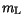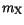References Si 0.328 0.19 0.98 0.55 0.49 0.16 [90,86,85] Ge 0.222 0.42 0.082 1.64 0.28 0.29 0.04 [90,86,85,94] GaAs 0.067 0.471 0.229 1.987 0.49 0.49 0.08 [117,86,115,150] AlAs 0.15 0.38 0.19 1.5 0.81 0.76 0.16 [108,71] InAs 0.023 0.64 0.271 3.619 0.41 0.41 0.025 [86,85,88,94] InP 0.077 0.462 0.273 1.321 0.64 0.6 0.12 [108,71,92,94] GaP 0.15 0.5 0.25 1.993 0.6 0.79 0.14 [85,94,73,91]

The temperature dependence of the relative carrier masses is well studied in the case of Si (see  and references therein). However, for III-V materials such data are scattering or missing and the effect is often neglected. A linear temperature dependence (3.88) is sufficient to model the relative electron masses for all materials . A quadratic temperature dependence model (3.89) is introduced for the relative hole masses, as the simplest expression sufficient to fit well the data for Si. Table 3.17 summarizes the values of the model parameters. The values ofshown in brackets are not taken into account for basic materials. They are used in the calculation of the relative electron masses in alloy materials.

Table 3.17: Parameter values for modeling the effective carrier masses
 Material Minimum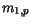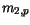Si X 0.321 0.009 0.55 0.6 -0.1 Ge L (X) 0.2158 (0.42) 0.0068 0.2915 0.1 GaAs(X) 0.067 (0.471) -0.0036 0.49 AlAs X () 0.38 (0.15) -0.0036 0.8 InAs(X) 0.0237 (0.64) -0.0027 0.41 InP(X) 0.077 (0.462) -0.004 0.64 GaP X () 0.5 (0.15) 0.6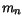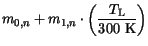(3.88)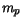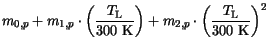(3.89)

For alloy materials a temperature dependence is introduced first. Then a quadratic interpolation of the masses is used as a function of the material composition of an alloy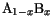.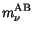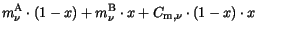(3.90)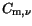are bowing parameters summarized in Table 3.18.

Table 3.18: Bowing parameter values for modeling the effective carrier masses
 Material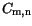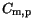Reported Range References SiGe -0.183 -0.096 AlGaAs 0.0 0.0 [110,93] InGaAs -0.012 -0.03 0.038-0.044 [152,93,153] x=0.53 InAlAs 0.0 0.0 0.070-0.083 [102,93,111,153] x=0.52 InAsP 0.0 0.0 GaAsP 0.0 0.0 InGaP 0.0 0.0 0.092-0.118 [103,154,155,156] x=0.5

For materials where the bandgap changes between direct and indirect the relative electron mass of the lowest band is considered. Such examples are AlGaAs (Fig. 3.29), InAlAs (Fig. 3.30), InGaP (Fig. 3.31), and GaAsP. For other materials, such as InGaAs (Fig. 3.32) and InAsP only thevalley masses are considered. For SiGe a strained bandgap without X-to-L transition is assumed and only the X-valley masses are used in the interpolation.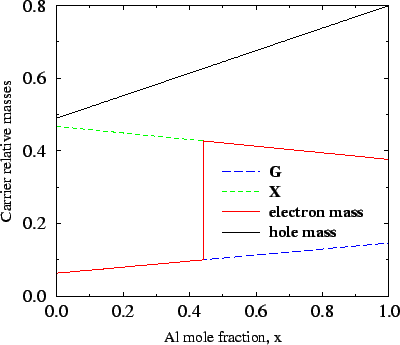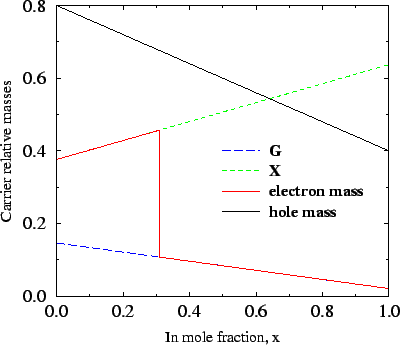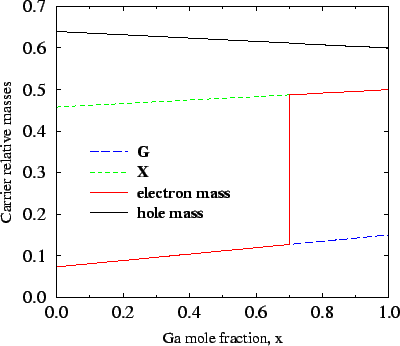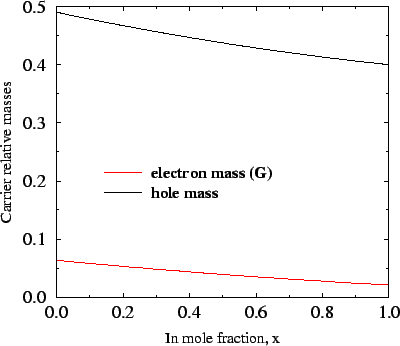Next: 3.3.5 Effective Density of Up: 3.3 Band-Structure Previous: 3.3.3 Bandgap Narrowing
Vassil Palankovski
2001-02-28